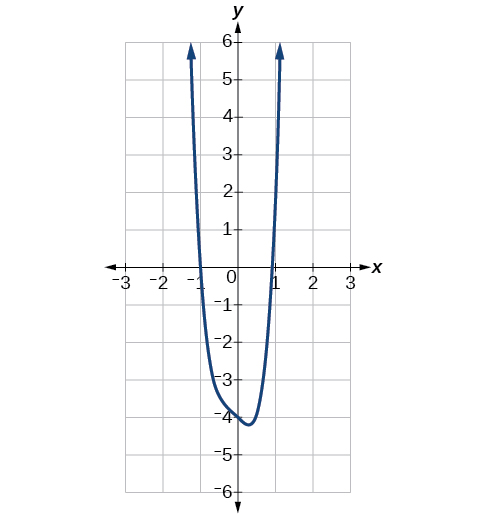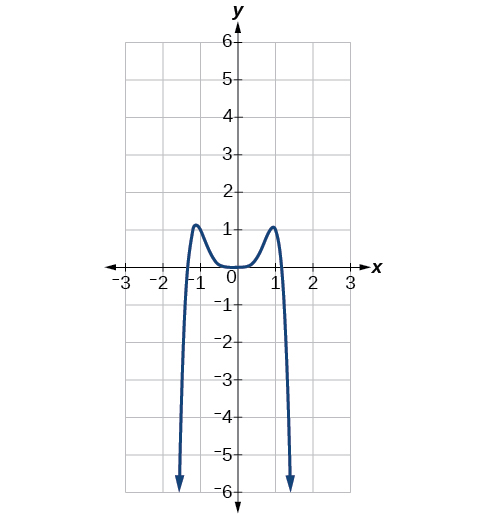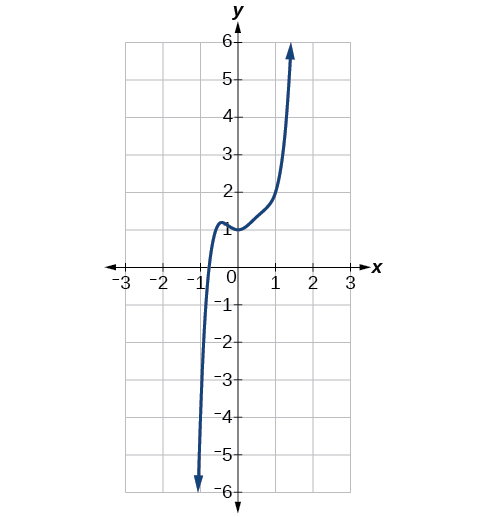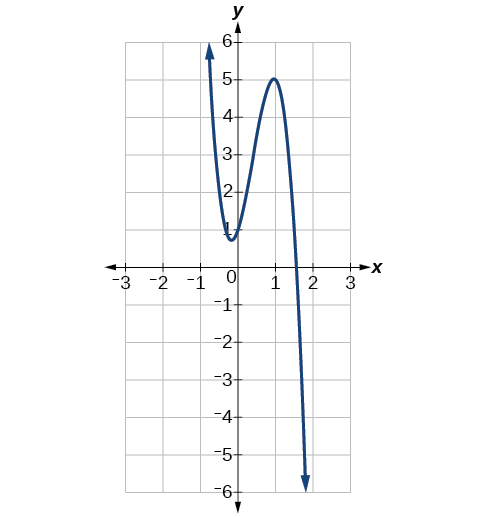# 5.2 Power functions and polynomial functions  (Page 4/19)

 Page 4 / 19

## Identifying the degree and leading coefficient of a polynomial function

Identify the degree, leading term, and leading coefficient of the following polynomial functions.

$\begin{array}{ccc}\hfill f\left(x\right)& =& 3+2{x}^{2}-4{x}^{3}\hfill \\ \hfill g\left(t\right)& =& 5{t}^{2}-2{t}^{3}+7t\hfill \\ h\left(p\right)\hfill & =& 6p-{p}^{3}-2\hfill \end{array}$

For the function $\text{\hspace{0.17em}}f\left(x\right),\text{\hspace{0.17em}}$ the highest power of $\text{\hspace{0.17em}}x\text{\hspace{0.17em}}$ is 3, so the degree is 3. The leading term is the term containing that degree, $\text{\hspace{0.17em}}-4{x}^{3}.\text{\hspace{0.17em}}$ The leading coefficient is the coefficient of that term, $\text{\hspace{0.17em}}-4.$

For the function $\text{\hspace{0.17em}}g\left(t\right),\text{\hspace{0.17em}}$ the highest power of $\text{\hspace{0.17em}}t\text{\hspace{0.17em}}$ is $\text{\hspace{0.17em}}5,\text{\hspace{0.17em}}$ so the degree is $\text{\hspace{0.17em}}5.\text{\hspace{0.17em}}$ The leading term is the term containing that degree, $\text{\hspace{0.17em}}5{t}^{5}.\text{\hspace{0.17em}}$ The leading coefficient is the coefficient of that term, $\text{\hspace{0.17em}}5.$

For the function $\text{\hspace{0.17em}}h\left(p\right),\text{\hspace{0.17em}}$ the highest power of $\text{\hspace{0.17em}}p\text{\hspace{0.17em}}$ is $\text{\hspace{0.17em}}3,\text{\hspace{0.17em}}$ so the degree is $\text{\hspace{0.17em}}3.\text{\hspace{0.17em}}$ The leading term is the term containing that degree, $\text{\hspace{0.17em}}-{p}^{3}.\text{\hspace{0.17em}}$ The leading coefficient is the coefficient of that term, $\text{\hspace{0.17em}}-1.$

Identify the degree, leading term, and leading coefficient of the polynomial $\text{\hspace{0.17em}}f\left(x\right)=4{x}^{2}-{x}^{6}+2x-6.$

The degree is 6. The leading term is $\text{\hspace{0.17em}}-{x}^{6}.\text{\hspace{0.17em}}$ The leading coefficient is $\text{\hspace{0.17em}}-1.$

## Identifying end behavior of polynomial functions

Knowing the degree of a polynomial function is useful in helping us predict its end behavior. To determine its end behavior, look at the leading term of the polynomial function. Because the power of the leading term is the highest, that term will grow significantly faster than the other terms as $\text{\hspace{0.17em}}x\text{\hspace{0.17em}}$ gets very large or very small, so its behavior will dominate the graph. For any polynomial, the end behavior of the polynomial will match the end behavior of the power function consisting of the leading term. See [link] .

Polynomial Function Leading Term Graph of Polynomial Function
$f\left(x\right)=5{x}^{4}+2{x}^{3}-x-4$ $5{x}^{4}$$f\left(x\right)=-2{x}^{6}-{x}^{5}+3{x}^{4}+{x}^{3}$ $-2{x}^{6}$$f\left(x\right)=3{x}^{5}-4{x}^{4}+2{x}^{2}+1$ $3{x}^{5}$$f\left(x\right)=-6{x}^{3}+7{x}^{2}+3x+1$ $-6{x}^{3}$## Identifying end behavior and degree of a polynomial function

Describe the end behavior and determine a possible degree of the polynomial function in [link] .

As the input values $\text{\hspace{0.17em}}x\text{\hspace{0.17em}}$ get very large, the output values $\text{\hspace{0.17em}}f\left(x\right)\text{\hspace{0.17em}}$ increase without bound. As the input values $\text{\hspace{0.17em}}x\text{\hspace{0.17em}}$ get very small, the output values $\text{\hspace{0.17em}}f\left(x\right)\text{\hspace{0.17em}}$ decrease without bound. We can describe the end behavior symbolically by writing

In words, we could say that as $\text{\hspace{0.17em}}x\text{\hspace{0.17em}}$ values approach infinity, the function values approach infinity, and as $\text{\hspace{0.17em}}x\text{\hspace{0.17em}}$ values approach negative infinity, the function values approach negative infinity.

We can tell this graph has the shape of an odd degree power function that has not been reflected, so the degree of the polynomial creating this graph must be odd and the leading coefficient must be positive.

Describe the end behavior, and determine a possible degree of the polynomial function in [link] .

As It has the shape of an even degree power function with a negative coefficient.

## Identifying end behavior and degree of a polynomial function

Given the function $\text{\hspace{0.17em}}f\left(x\right)=-3{x}^{2}\left(x-1\right)\left(x+4\right),\text{\hspace{0.17em}}$ express the function as a polynomial in general form, and determine the leading term, degree, and end behavior of the function.

Obtain the general form by expanding the given expression for $\text{\hspace{0.17em}}f\left(x\right).$

$\begin{array}{ccc}\hfill f\left(x\right)& =& -3{x}^{2}\left(x-1\right)\left(x+4\right)\hfill \\ & =& -3{x}^{2}\left({x}^{2}+3x-4\right)\hfill \\ & =& -3{x}^{4}-9{x}^{3}+12{x}^{2}\hfill \end{array}$

The general form is $\text{\hspace{0.17em}}f\left(x\right)=-3{x}^{4}-9{x}^{3}+12{x}^{2}.\text{\hspace{0.17em}}$ The leading term is $\text{\hspace{0.17em}}-3{x}^{4};\text{\hspace{0.17em}}$ therefore, the degree of the polynomial is 4. The degree is even (4) and the leading coefficient is negative (–3), so the end behavior is

#### Questions & Answers

write down the polynomial function with root 1/3,2,-3 with solution
if A and B are subspaces of V prove that (A+B)/B=A/(A-B)
write down the value of each of the following in surd form a)cos(-65°) b)sin(-180°)c)tan(225°)d)tan(135°)
Prove that (sinA/1-cosA - 1-cosA/sinA) (cosA/1-sinA - 1-sinA/cosA) = 4
what is the answer to dividing negative index
In a triangle ABC prove that. (b+c)cosA+(c+a)cosB+(a+b)cisC=a+b+c.
give me the waec 2019 questions
the polar co-ordinate of the point (-1, -1)
prove the identites sin x ( 1+ tan x )+ cos x ( 1+ cot x )= sec x + cosec x
tanh`(x-iy) =A+iB, find A and B
B=Ai-itan(hx-hiy)
Rukmini
what is the addition of 101011 with 101010
If those numbers are binary, it's 1010101. If they are base 10, it's 202021.
Jack
extra power 4 minus 5 x cube + 7 x square minus 5 x + 1 equal to zero
the gradient function of a curve is 2x+4 and the curve passes through point (1,4) find the equation of the curve
1+cos²A/cos²A=2cosec²A-1
test for convergence the series 1+x/2+2!/9x3ByByByBy MldelatteByBy Rhodes